﻿ 北斗单历元定位误差及去噪特性分析

# 北斗单历元定位误差及去噪特性分析Analysis of Positioning Error and De-Noising Characteristic of Beidou Single Epoch

Abstract: The error characteristics of Beidou satellite base line single epoch time series are analyzed. We believe that the influence of periodic error and high-frequency noise is mainly included in the time series. In this paper, the high frequency noise in time series is filtered by means of median filtering and wavelet analysis. The experimental results show that the high frequency noise is close to the normal distribution characteristic, which can be classified as accidental error and can be better suppressed by filtering. The wavelet analysis is more suitable for Beidou single epoch de-noising.

1. 引言

2. 北斗基线时间序列的误差特性

3. 去噪方法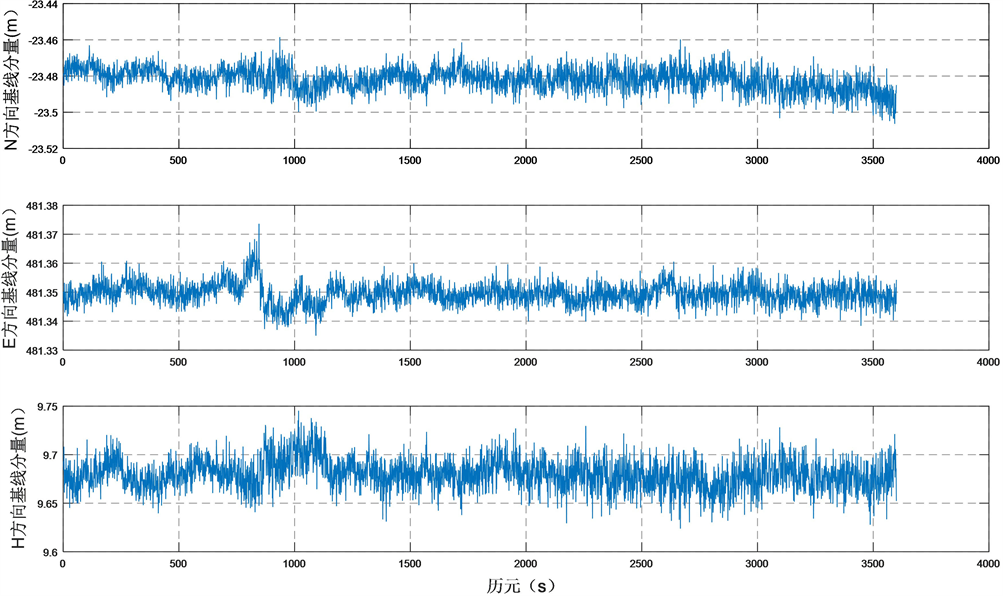Figure 1. BDS baseline solutions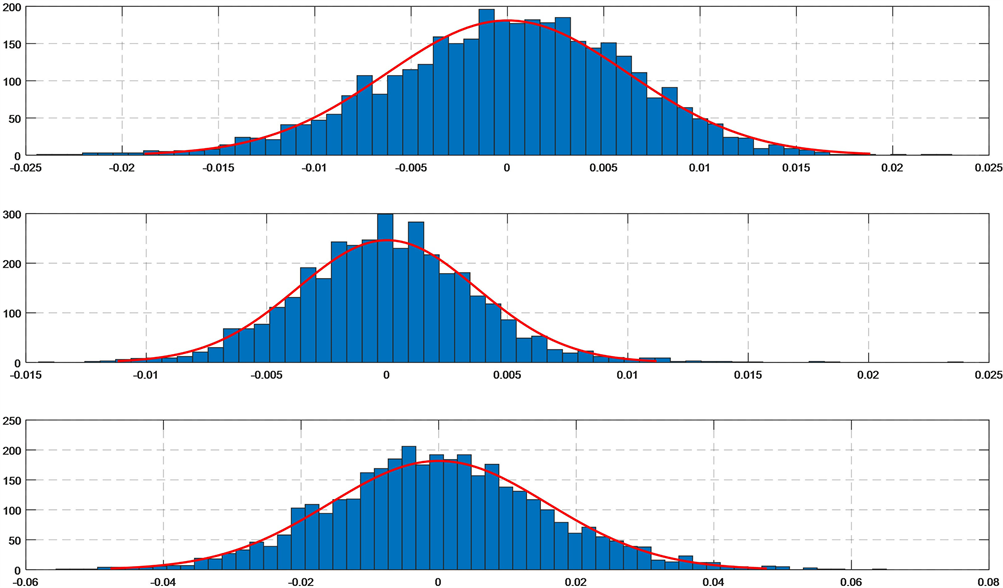Figure 2. BDS baseline residual error distribution

1) 中值滤波

3600个滤波残差的统计特性如图4所示。从图4中我们可以看出，过滤残差近似服从正态分布，因此中值滤波的过滤部分属于随机偶然误差。

2) 小波去噪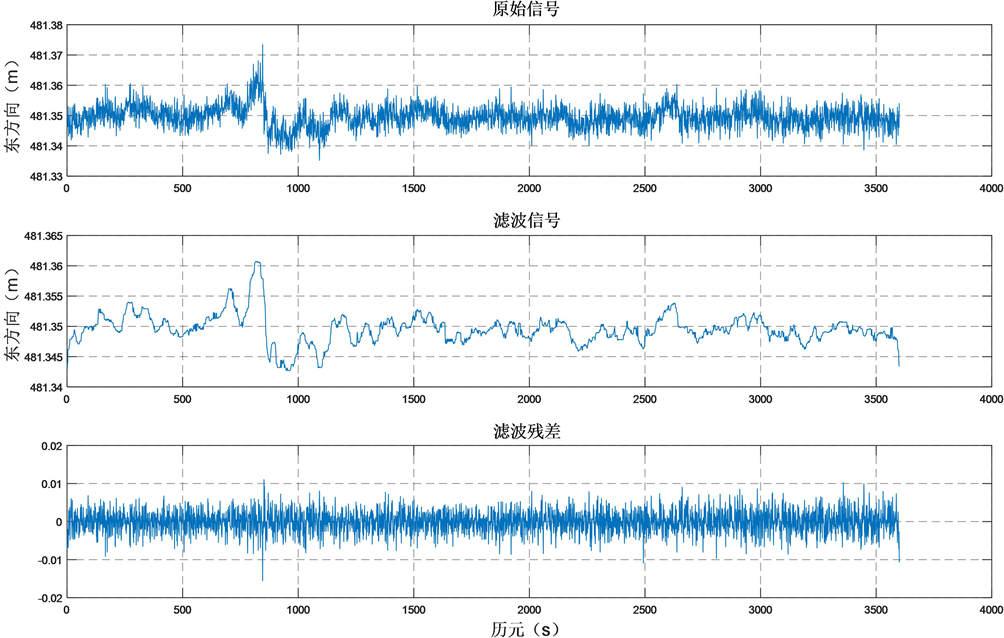Figure 3. Baseline 2021~2951 east component and its high frequency noise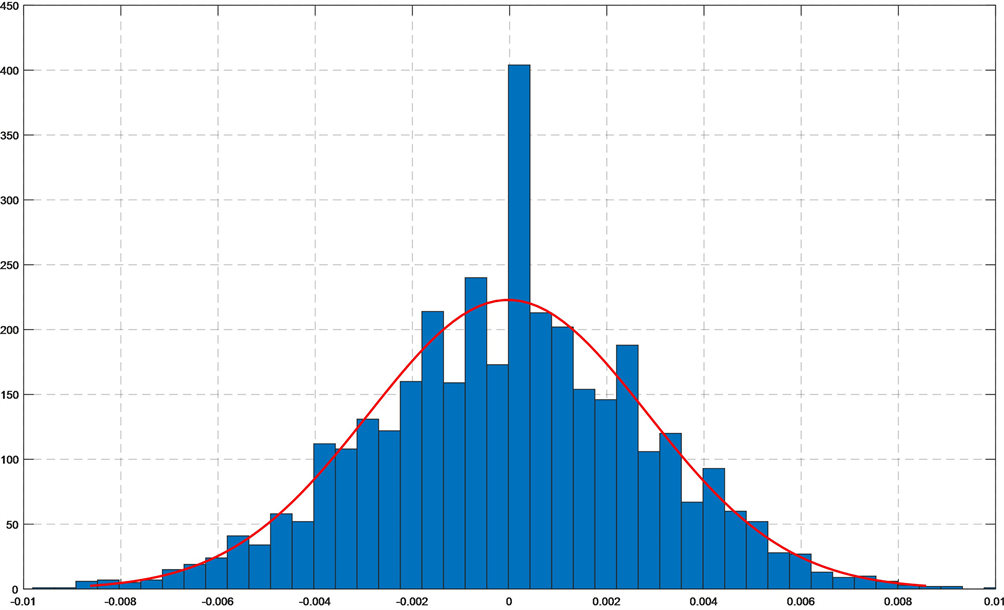Figure 4. The residual error statistic

a) 分解过程：首先根据信号的特性和波形选择合适的小波，再对需要处理的信号进行多层分解，然后通过小波变换，得到小波变换的系数。

b) 阈值过程：根据公式计算小波阈值，得到小波阈值后再选择合适的阈值对分解后的各层系数进行降噪处理。

c) 重构过程：对降噪处理后的结果进行小波逆变换，将去噪后的信号进行恢复。

4. 去噪效果评价

1) 均方根误差

$\text{RMSE}=\sqrt{\frac{{\left(x\left(i\right)-\stackrel{˜}{x}\left(i\right)\right)}^{2}}{n}}$ (1)

2) 信噪比

$\text{SNR}=10\mathrm{lg}\left[\frac{{\sum }_{i=1}^{n}x{\left(i\right)}^{2}}{{\sum }_{i=1}^{n}{\left(x\left(i\right)-\stackrel{˜}{x}\left(i\right)\right)}^{2}}\right]$ (2)

3) 平滑度

$r=\frac{{\sum }_{i=1}^{n-1}{\left[\stackrel{˜}{x}\left(i+1\right)-\stackrel{˜}{x}\left(i\right)\right]}^{2}}{{\sum }_{i=1}^{n-1}{\left[x\left(i+1\right)-x\left(i\right)\right]}^{2}}$ (3)

4) 相关系数

$R=\frac{{\sum }_{i=1}^{n}\left(x\left(i\right)-\stackrel{¯}{x\left(i\right)}\right)\left(\stackrel{˜}{x}\left(i\right)-\stackrel{¯}{\stackrel{˜}{x}\left(i\right)}\right)}{\sqrt{{\sum }_{i=1}^{n}{\left(x\left(i\right)-\stackrel{¯}{x\left(i\right)}\right)}^{2}\cdot {\sum }_{i=1}^{n}{\left(\stackrel{˜}{x}\left(i\right)-\stackrel{¯}{\stackrel{˜}{x}\left(i\right)}\right)}^{2}}}$ (4)

5. 去噪结果分析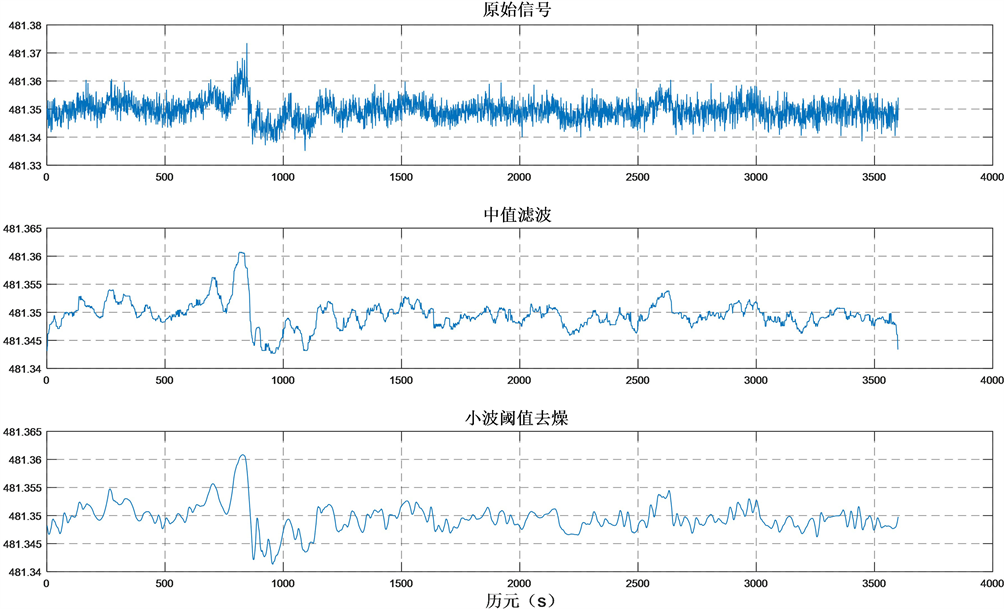Figure 5. Comparison of de-noising resultsTable 1. Filtering results of two methods (east direction)Table 2. Filtering results of two methods (north direction)Table 3. Filtering results of two methods (height direction)

6. 结论

NOTES

*通讯作者。

 中国卫星导航系统管理办公室. 北斗卫星导航系统空间信号接口控制文件公开服务信号(2.1版) [Z]. 北京, 2016.

 刘思慧, 樊婧琦, 欧钢, 等. 多径效应的动态特性对码跟踪环路的影响分析[J]. 测绘学报, 2011(S1): 89-94.

 陆亚峰, 楼立志, 马绪瀛, 等. 北斗伪距多路径分析及单点定位解算[J]. 矿山测量, 2013(4): 18-21 + 24.

 韩晓飞, 马绪瀛, 丁晓光, 等. 北斗单历元基线解算与恒星日滤波算法[J]. 测绘通报, 2015(4): 5-9.

 王建军, 马绪瀛. 北斗系统多路径误差特性分析与消除策略[J]. 测绘科学, 2016(1): 18-22.

 丁文. 基于小波和Kalman滤波的GPS数据去噪方法研究[D]: [硕士学位论文]. 沈阳: 东北大学, 2009.

 崔红超. 小波分析在GPS数据处理中的应用研究[D]: [硕士学位论文]. 南昌: 东华理工大学, 2013.

 李宗春, 邓勇, 张冠宇, 等. 变形测量异常数据处理中小波变换最佳级数的确定[J]. 武汉大学学报(信息科学版), 2011, 36(3): 285-288.

 陶珂, 朱建军. 多指标融合的小波去噪最佳分解尺度选择方法[J]. 测绘学报, 2012, 41(5): 749-755.

 朱建军, 章浙涛, 匡翠林, 等. 一种可靠的小波去噪质量评价指标[J]. 武汉大学学报(信息科学版), 2015, 40(5): 688-694.

 胡薛毅, 黄声享, 庞辉. 变形监测数据小波去噪分解尺度确定方法[J]. 测绘地理信息, 2017, 42(2): 44-48.

Top